# Invariant differential operator

(diff) ← Older revision | Latest revision (diff) | Newer revision → (diff)
A differential operator that does not change its form under certain transformations of the space on which it is defined. For example, ifis a partial differential operator written out in some coordinate system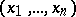, if,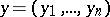, is some transformation of coordinates inducing a corresponding mapping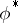in the set of functions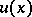(each functionis associated in a natural way with the function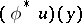) and if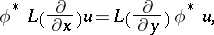where the operatoron the right-hand side is expressed in terms of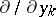in the same way as the operatoron the left-hand side is expressed in terms of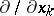, thenis said to be invariant under the transformation(oris said to commute with the operator transformation). The most important case is when a differential operator is invariant under a family of transformations forming a group. The definition of an invariant differential operator becomes substantially more complicated if one considers a system of functions transformed by some representation of this group of transformations. The invariant differential operators related to the Lorentz group and the orthogonal group (the wave operator, the Klein–Gordon and Laplace operators, etc.) play an important role in mathematical physics. In analysis on differentiable manifolds one extensively uses the operator of exterior differentiation, which is invariant under diffeomorphisms, and the operator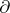, metrically dual to it, which is invariant under smooth transformations that preserve the metric tensor. In the theory of Lie groups, the so-called left- and right-invariant operators under the corresponding shifts on the group are of great importance.